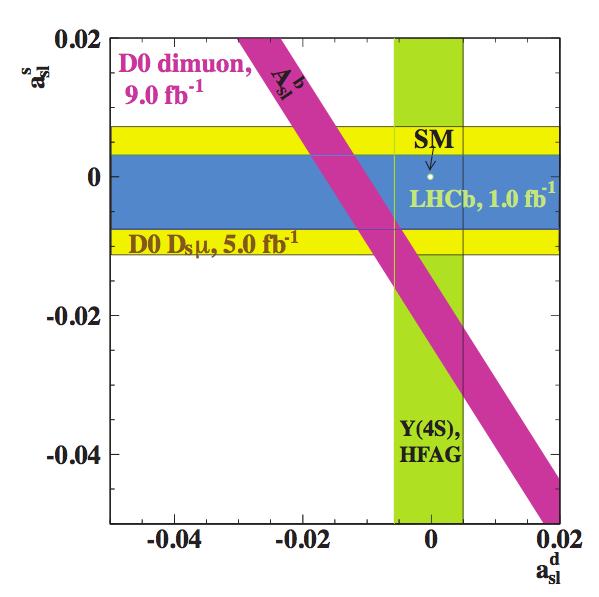# LHCb Results

## Selected Results from LHCb Link

Here is one of the latest measurements from LHCb, giving you a sense of what kind of results are obtained from current particle physics experiments. Don’t worry about understanding the details–it takes even experts quite a while to understand them fully. Remember: this is basic science so results may seem hard to grasp but nevertheless they are important and have far-reaching implications for understanding the way the universe works!

### Measurement of Semileptonic CP Asymmetry LinkHere is a plot showing one of the most recent results from LHCb. This is a measurement of what is called the “Semileptonic CP Asymmetry,” which is the difference between the number of particles that are produced in a particular decay of a B meson vs the number that is produced in the decay of the anti-B meson. This effect is due to what is called CP-violation, which is bound up with the whole idea of particles and their antiparticles. This measurement gives us a clue as to why there is more matter than antimatter in the universe.

This figure illustrates the measurements of semileptonic CP asymmetries. The bands correspond to the central measured values ±1 standard deviation, defined as the sum in quadrature of the statistical and systematic errors. The yellow dot at (0,0) on the plot is the Standard Model prediction for this asymmetry, and the blue band is the LHCb result with errors included. This overlapping of the blue band with the SM prediction simply means that currently the asymmetry is consistent with zero.

• More “Plain English” summaries by our group members can be found  here.
• Or, see the LHCb public webpage here.

< PREV | NEXT >